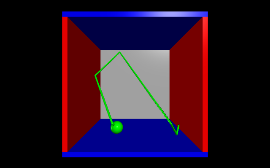GlowScript and VPython

From glowscript.org, "GlowScript is an easy-to-use, powerful environment for creating 3D animations and publishing them on the web. The design of GlowScript is based on that of VPython. Development of GlowScript was initiated by David Scherer and Bruce Sherwood in the summer of 2011."Just like with GlowScript, you don't need to import any extra modules to get started. Visit the GlowScript Help pages for information on what types of objects, events, and other graphing functionality is available.

Here is a quick example to help get you started.

GlowScript 1.1 VPython
scene.caption.text("""Right button drag or Ctrl-drag to rotate "camera" to view scene.
Middle button or Alt-drag to drag up or down to zoom in or out.
On a two-button mouse, middle is left + right.
Touch screen: pinch/extend to zoom, swipe or two-finger rotate.""")

side = 4.0
thk = 0.3
s2 = 2*side - thk
s3 = 2*side + thk

wallR = box (pos=vector( side, 0, 0), size=vector(thk, s2, s3),  color = color.red)
wallL = box (pos=vector(-side, 0, 0), size=vector(thk, s2, s3),  color = color.red)
wallB = box (pos=vector(0, -side, 0), size=vector(s3, thk, s3),  color = color.blue)
wallT = box (pos=vector(0,  side, 0), size=vector(s3, thk, s3),  color = color.blue)
wallBK = box(pos=vector(0, 0, -side), size=vector(s2, s2, thk), color = vector(0.7,0.7,0.7))

ball = sphere (color = color.green, radius = 0.4, make_trail=True, retain=200)
ball.mass = 1.0
ball.p = vector (-0.15, -0.23, +0.27)

side = side - thk*0.5 - ball.radius

dt = 0.3
while True:
rate(200)
ball.pos = ball.pos + (ball.p/ball.mass)*dt
if not (side > ball.pos.x > -side):
ball.p.x = -ball.p.x
if not (side > ball.pos.y > -side):
ball.p.y = -ball.p.y
if not (side > ball.pos.z > -side):
ball.p.z = -ball.p.z